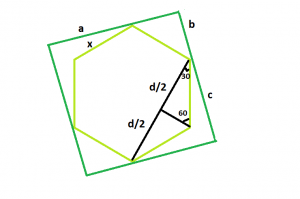# Largest hexagon that can be inscribed within a square

Given side of a square a, the task is to find the side of the largest hexagon that can be inscribed within the given square.

Examples:

Input: a = 6
Output: 3.1056

Input: a = 8
Output: 4.1408

## Recommended: Please try your approach on {IDE} first, before moving on to the solution.Approach:: Let, the side of the hexagon be x and assume that the side of the square, a gets divided into smaller length b & bigger length c i.e. a = b + c
Now from the figure, we see,

b2 + b2 = x2 which gives b = x / √2
Now again, d / (2 * x) = cos(30) = √3 / 2 i.e. d = x√3
And, c2 + c2 = d2 which gives c = d / √2 = x√3 / √2
Since, a = b + c. So, a = x / √2 + x√3 / √2 = ((1 + √3) / √2) * x = 1.932 * x
So, side of the hexagon, x = 0.5176 * a

Below is the implementation of the above approach:

## C++

 `// C++ Program to find the biggest hexagon which ` `// can be inscribed within the given square ` `#include ` `using` `namespace` `std; ` ` `  `// Function to return the side ` `// of the hexagon ` `float` `hexagonside(``float` `a) ` `{ ` ` `  `    ``// Side cannot be negative ` `    ``if` `(a < 0) ` `        ``return` `-1; ` ` `  `    ``// Side of the hexagon ` `    ``float` `x = 0.5176 * a; ` `    ``return` `x; ` `} ` ` `  `// Driver code ` `int` `main() ` `{ ` `    ``float` `a = 6; ` `    ``cout << hexagonside(a) << endl; ` `    ``return` `0; ` `} `

## Java

 `// Java  Program to find the biggest hexagon which ` `// can be inscribed within the given square ` ` `  `import` `java.io.*; ` ` `  `class` `GFG { ` `     `  `// Function to return the side ` `// of the hexagon ` `static` `double` `hexagonside(``double` `a) ` `{ ` ` `  `    ``// Side cannot be negative ` `    ``if` `(a < ``0``) ` `        ``return` `-``1``; ` ` `  `    ``// Side of the hexagon ` `    ``double` `x = (``0.5176` `* a); ` `    ``return` `x; ` `} ` ` `  `// Driver code ` `    ``public` `static` `void` `main (String[] args) { ` ` `  `        ``double` `a = ``6``; ` `        ``System.out.println (hexagonside(a)); ` `    ``} ` `//This code is contributed by ajit.     ` `} `

## Python 3

 `# Python 3 Program to find the biggest  ` `# hexagon which can be inscribed within ` `# the given square ` ` `  `# Function to return the side ` `# of the hexagon ` `def` `hexagonside(a): ` ` `  `    ``# Side cannot be negative ` `    ``if` `(a < ``0``): ` `        ``return` `-``1``; ` ` `  `    ``# Side of the hexagon ` `    ``x ``=` `0.5176` `*` `a; ` `    ``return` `x; ` ` `  `# Driver code ` `a ``=` `6``; ` `print``(hexagonside(a)); ` ` `  `# This code is contributed  ` `# by Akanksha Rai `

## C#

 `// C# Program to find the biggest hexagon which  ` `// can be inscribed within the given square  ` `using` `System; ` ` `  `class` `GFG ` `{  ` `         `  `// Function to return the side  ` `// of the hexagon  ` `static` `double` `hexagonside(``double` `a)  ` `{  ` ` `  `    ``// Side cannot be negative  ` `    ``if` `(a < 0)  ` `        ``return` `-1;  ` ` `  `    ``// Side of the hexagon  ` `    ``double` `x = (0.5176 * a);  ` `    ``return` `x;  ` `}  ` ` `  `// Driver code  ` `public` `static` `void` `Main ()  ` `{  ` `    ``double` `a = 6;  ` `    ``Console.WriteLine(hexagonside(a));  ` `}  ` `}  ` ` `  `// This code is contributed by Ryuga.  `

## PHP

 ` `

Output:

```3.1056
```

Attention reader! Don’t stop learning now. Get hold of all the important DSA concepts with the DSA Self Paced Course at a student-friendly price and become industry ready.

My Personal Notes arrow_drop_upCheck out this Author's contributed articles.

If you like GeeksforGeeks and would like to contribute, you can also write an article using contribute.geeksforgeeks.org or mail your article to contribute@geeksforgeeks.org. See your article appearing on the GeeksforGeeks main page and help other Geeks.

Please Improve this article if you find anything incorrect by clicking on the "Improve Article" button below.

Improved By : jit_t, AnkitRai01, Akanksha_Rai